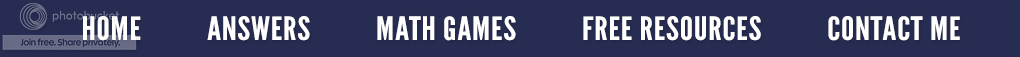When Dividing, Zero Is No Hero

Have you ever wondered why we can't divide by zero?  I remembering asking that long ago in a math class, and the teacher's response was, "Because we just can't!"  I just love it when things are so clearly explained to me. So instead of a rote answer, let's investigate the question step-by-step.

The first question we need to answer is what does a does division mean?  Let's use the example problem on the right.

1. The 6 inside the box means we have six items such as balls. (dividend)
2. The number 2 outside the box (divisor) tells us we want to put or separate the six balls into two groups.
3. The question is, “How many balls will be in each group?”
4. The answer is, “Three balls will be in each of the two groups.” (quotient)

Using the sequence above, let's look at another problem, only this time let's divide by zero.
1. The 6 inside the box means we have six items like balls. (dividend)
2. The number 0 outside the box (divisor) tells us we want to put or separate the balls into groups into no groups.
3. The question is, “How many balls can we put into no groups?”
4. The answer is, “If there are no groups, we cannot put the balls into a group.”
5. Therefore, we cannot divide by zero because we will always have zero groups (or nothing) in which to put things. You can’t put something into nothing.
Let’s look at dividing by zero a different way. We know that division is the inverse (opposite) of multiplication; so………..
1. In the problem 12 ÷ 3 = 4.  This means we can divide 12 into three equal groups with four in each group.
2. Accordingly, 4 × 3 = 12.  Four groups with three in each group equals 12 things.
So returning to our problem of six divided by zero.....
1. If 6 ÷ 0 = 0.......
2. Then 0 × 0 should equal 6, but it doesn’t; it equals 0. So in this situation, we cannot divide by zero and get the answer of six.

We also know that multiplication is repeated addition; so in the first problem of 12 ÷ 3, if we add three groups of 4 together, we should get a sum of 12. 4 + 4 + 4 = 12

As a result, in the second example of 6 ÷ 0, if six zeros are added together, we should get the answer of 6. 0 + 0 + 0 + 0 + 0 + 0 = 0 However we don’t. We get 0 as the answer; so, again our answer is wrong.

It is apparent that how many groups of zero we have is not important because they will never add up to equal the right answer. We could have as many as one billion groups of zero, and the sum would still equal zero. So, it doesn't make sense to divide by zero since there will never be a good answer. As a result, in the Algebraic world, we say that when we divide by zero, the answer is undefined. I guess that is the same as saying, "You can't divide by zero," but now at least you know why.Free - Why We Can't Divide by Zero